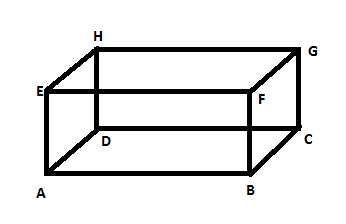Courses
Courses for Kids
Free study material
Free LIVE classes
MoreLIVE
Join Vedantu’s FREE Mastercalss

# How many edges are there in a cuboid? A. 4B. 5C. 6D. 12Verified
362.7k+ views
Hint: Here we can use Euler’s formula. The number of faces, the number of vertices and the number of edges of a polyhedron are related by the following formula:

Number of faces + number of vertices = number of edges + 2.

In this problem we have to find out how many edges are there in a cuboid. So, let us first draw a cuboid. We know that a cuboid is a solid figure which has six rectangular faces at right angles to each other.First we have to know about the vertex, edge and face of a solid figure.
A vertex is a point where two or more line segments meet. Basically a vertex is a corner. Plural of vertex is vertices. So, in this picture the vertices are $A,B,C,D,E,F,G,H$ . So there are 8 vertices in a cuboid.
A face is a single flat surface of a solid object. Like here in this figure the faces are:$ABCD,EFGH,ABFE,DCGH,ADHE,BCGF$
So, there are 6 faces in a cuboid.
An edge is a line segment between two faces. For example here the line segment between the faces $ABCD$ and $DCGH$ is $DC$ .
Now, we know the Euler’s formula:
Number of faces + number of vertices = number of edges + 2
Let us denote the number of faces by $F$.
Number of vertices by $V$.
Number of edges by $E$.
Therefore, $F+V=E+2...........(1)$
Here, the number of faces are 6. Numbers of vertices are 8. Let us put these values in (1).
\begin{align} & 6+8=E+2 \\ & \Rightarrow 14=E+2 \\ & \Rightarrow E=14-2 \\ & \Rightarrow E=12 \\ \end{align}
Therefore the numbers of edges are 12.
Hence option (e) is correct.

Note: We can count the edges from the picture also. Like the edges are $AB, BC, CD, DA, EF, FG, GH, HE, AE, DH, BF, CG$. So there are 12 edges.
Last updated date: 02nd Oct 2023
Total views: 362.7k
Views today: 7.62k